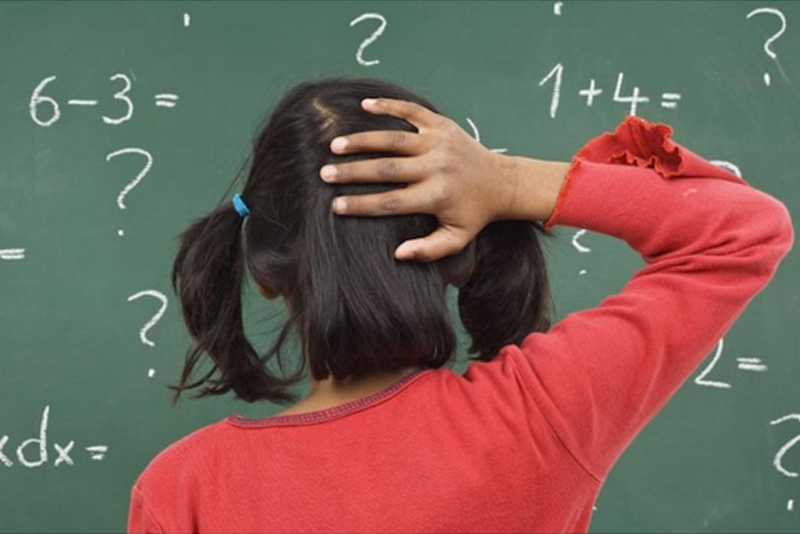# Learning mathematics for understandingLearning mathematics does not come as naturally as learning to speak. However, our brains have the capability of learning the numeracy content and understand the mathematical procedures, although it requires time and effort. The notion that some people are not able to do maths is a myth. It is generally understood that humans are born with the ability to appreciate the concept of number. Even little children can distinguish between two packs of sweets where one is larger than the other. As they grow, they acquire the ability to manipulate small quantities with accuracy and larger quantities through approximation. They are the base upon which more complex abilities are built.

The National Mathematics Advisory Panel of USA identified three types of knowledge required for learning knowledge: Factual, Procedural and Conceptual.

Factual knowledge can be described as the basic information that students must be acquainted with. Factual knowledge consists of statements, definitions, and conventions.

‘2+3 = 5’ is a statement.

‘Even numbers are numbers which are exactly divisible by 2’ is a definition.

‘When calculating expressions (such as 5 + 3 X 4), multiplication need to be carried out before addition’ is a convention.

With factual knowledge, the answer is not calculated but just retrieved from memory. These are learnt through exposure, repetition, and commitment to memory. The automatic retrieval of these basic mathematical facts is critical to be proficient in the subject. When students have difficulty in learning arithmetic, it is mostly due to the hardship of retrieving basic facts.

Procedural knowledge is a series of steps that must be followed to solve mathematical problems. It deals with learning strategies involving task specific rules or a sequence of actions to reach a required goal. Consider the following example:

Solve    2(3x – 2) + 3( x + 1) = 17

6x – 4 + 3x + 3         = 17           (Remove brackets)

6x + 3x                    = 17 +4 – 3            (Variables on the left and constants on the right)

9x                            = 18           (simplify each side)

x                             = 2                        (Divide by the coefficient of the variable)

Perhaps the student may not have fully grasped the idea behind this procedure but followed the required algorithm.

Conceptual knowledge is the understanding of core principles and the relationships among them. The National Council of Teachers of Mathematics defines conceptual knowledge as follows.

“Students demonstrate conceptual understanding in mathematics when they provide evidence that they can recognize, label, and generate examples of concepts; use and interrelate models, diagrams, manipulatives, and varied representations of concepts; identify and apply principles; know and apply facts and definitions; compare, contrast, and integrate related concepts and principles; recognize, interpret, and apply the signs, symbols, and terms used to represent concepts. Conceptual understanding reflects a student’s ability to reason in settings involving the careful application of concept definitions, relations, or representations of either.”

There have been debates over whether it is better to teach conceptual or procedural understanding first. Some have argued that with sufficient practice of algorithms, students will gain a conceptual understanding of the procedures while others feel that with a good conceptual understanding the procedures to solve problems will follow automatically. The present understanding is that both can be taught simultaneously as students gain knowledge and understanding of one, it can then support the comprehension of the other.

The mathematical incompetence of students is often due to the absence of a connection between conceptual and procedural knowledge. When students fail to solve problems, many teachers explain details of the algorithms rather than addressing understanding. Conceptual understanding improves procedural knowledge more than procedural understanding improves conceptual knowledge. Therefore, mathematics teachers should concentrate more on conceptually based teaching environments and moving away from, but not eliminating, procedure-based learning environments. To illustrate the difference between the two when it comes to exercises, I give below a few examples.

1. Procedural: If you sleep 8 hours a day, what fraction of the day is spent sleeping?

Conceptual: “Many people spend 1/3 of the day sleeping”. Do you agree with this statement?

• Procedural:  Find the perimeter and the area of a rectangle with length 30 cm and width 20 cm.

Conceptual: Find the dimensions of a rectangle with perimeter 100 cm and area 600cm2.

• Procedural: Find the value of–Conceptual: Which is biggerorand by how much?

• Procedural: Find the roots of x2 – 2x – 15 = 0

Conceptual: x= 5 satisfies the equation x2 – ax -15 = 0. Find the value of ‘a’ and find other values for x that satisfy the equation, if any.

• Procedural: y =. Find y when x = -4, -2, 0, 2 and 4.

Conceptual: y =. Are there any values that x cannot take? What about y?

To create a climate that encourages understanding, the following features may be considered in a classroom situation.

• – Give importance to the ideas expressed by students. Respect for ideas is critical for a productive discussion.
• – Encourage student to student interaction to enable their confidence about their capability   of making sense of mathematics
• – Establish a culture of understanding that mistakes are good opportunities for learning.

Highlighting and using students’ ideas is crucial to promote mathematical proficiency. “It’s fine to work on any problem, so long as it generates interesting mathematics along the way – even if you don’t solve it at the end of the day” said Andrew Wiles.

R. N. A. de Silva

ndesilva@osc.lkThe author is a senior examiner for mathematics at the International Baccalaureate Organization and an educational consultant at the Overseas School of Colombo.